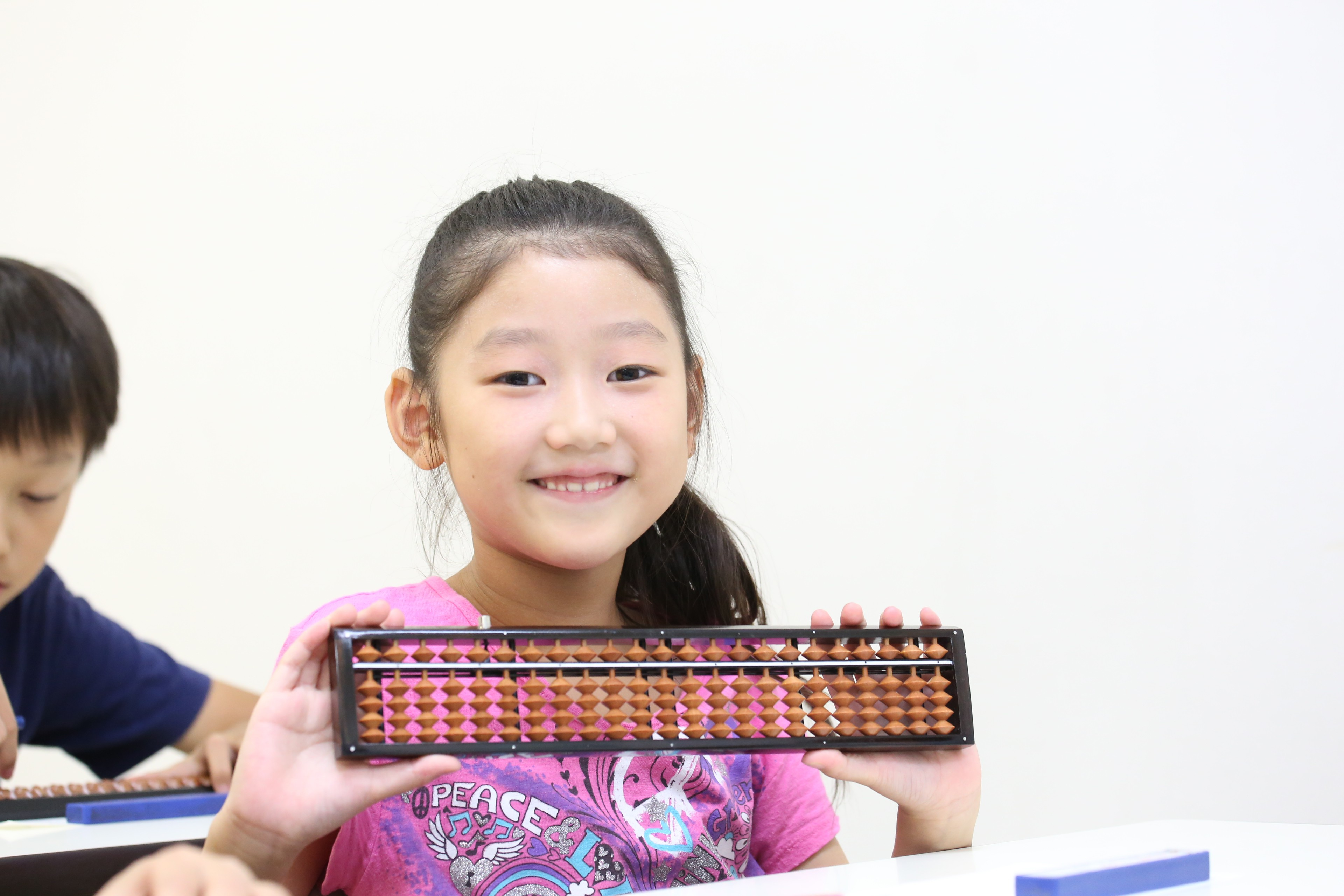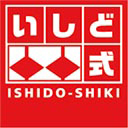Blog / ISHIDO-SHIKI / Sep 2020 - Abacus Math Examples for Students
Sep 2020 - Abacus Math Examples for Students

18 Sep,2020

# Sep 2020 - Abacus Math Examples for StudentsStudents who are interested to know more about abacus math examples can find this article useful to learn maths like addition, subtraction, multiplication, division, and even algebra too!

I'm the author of this article and I'm a qualified abacus teacher, answering questions from around the world online abacus and various questions from online-soroban.com students.

1. A Few Abacus Math Examples for You to Learn WithTeaching tools are made to help children learn in schools easily.

Children are supported while learning topics that may be complicated to understand.

Each tool must be selected carefully to not only teach the topic of interest but to help the kid to develop different skills as well.

Math learning is the same. Hence it is important for the teachers and educators to know what tools and sources use in order to provide the best future- and education possible.

## 1-1.What Are Some Benefits of Abacus Math Examples for Students?There are a variety of different math skills that adults are expected to have so that they can use it in different life situations, skills that – although in simpler ways – preschool and elementary-aged children are also expected to master.

The use of the abacus in math lessons facilitates the learning of those abilities, improving the approach towards math.

The soroban, as a teaching tool, gives kids a new point of view of math at the moment of learn arithmetic operations (a math skill that encompasses addition, subtraction, multiplication, and division); instead of just write the numbers and operations on paper, kids could play and experiment with the object of learning, using the beads to represent the numbers and its value, giving their complete attention to the mathematical tool on their hands to be able to resolve the problem.

Problem-solving abilities are crucial to success in both academic and day-to-day situations, situations that rely on higher cognitive processes.

Its use also potentiates the development of math reasoning (the comprehension, recognition and use of the different math skills) and math cardinality (the understanding that the last number used to count a group of objects represents how many are in the group).
If the use of the abacus is adequate, and it's given enough time for its practice, incredible results are achieved since they allow great speed in the realization of mental math.

## 1-2.Are There a Few Abacus Math Examples for School?But what is mental math? And why is it important for it to be developed in childhood?

Mental math is the ability to calculate and get correct answers without the use of a pencil and paper, or any other tool.

The constant use of the soroban will help to improve the use of mental math in the child, this is done because kids will eventually learn to project the abacus in their mind, therefore learning to calculate mathematical problems using the principles of the soroban, without the need of the physical tool.

However, there's more than the single development of mental maths because they'll also be undergoing the process of developing other aspects of the brain.

When the kids have acquired the ability to use mental math and mental abacus, this is actually the result of having developed the abilities of concentration, memory, visualization, and observation – key in math operations.

The practice of mental calculation boosts learning capabilities; while math skills are associated with the left side of the brain, mental math stimulates and focuses on the right side, which is responsible for visualization and creativity.

Solving math problems mentally requires to think of creative solutions and visualize numbers, involving the right brain.

## 1-3. What Are Some Abacus Math Examples for Education?But for this to happen teachers need first to understand the correct use of the soroban, and how this should be used to solve math problems and questions.

There are different approaches to teaching the use of the soroban to solve math questions, but since one single method might not work effectively for all students, teachers should be familiar with several methods.

The most used approaches in teaching are the logic method and the counting method.

The logic method focuses on understanding the what and why of the steps in solving a problem on the abacus.

It requires for the kid to know the partners of the numbers up to ten (5=2+3, 5=1+4), the teacher must verbalize the steps and reasons while the student works on the problem.

This approach helps students who can follow the explanation and understand the logical concepts involved.

Example: “The problem is 3+4. What number comes first? The answer is 3.

Set 3. Can we set 4 directly?

No. What is the smallest amount that can be set that is greater than 4?

The answer is 5. Set the 5 bead. How many more is 5 than 4?

The counting method needs for the student to count each bead as it’s added or subtracted, moving from the unit beads to the five beads (but only counting one for all beads).

This approach could be appropriate for young kids who would understand better a manipulative process, without having to focus on each individual step.

With Online Soroban's ISHIDO-SHIKI Japanese abacus, students will be able to understand these mathematical terms easily.

Overall
By understanding these approaches, your children will be able to benefit from the use of the soroban.

Primarily, they get better abilities in calculating compared to those kids without the knowledge of mental arithmetic; and secondary, it increases all their analytical and math abilities previously discussed, promoting patience and observatory skills as well.

Another benefit is that it helps to develop long term memory – when kids understand how to use the calculating device, they learn how to address problems and associate the steps to solve them with the desired solution; helping the kid to have a positive attitude towards math.

With the constant practice with the soroban, kids will be able to develop all the benefits associated with mental math, giving them access to various skills that will be of great help in the near future.

Same categoryLet's learning ISHIDO-SHIKI